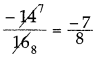# DAV Class 7 Maths Chapter 2 Worksheet 6 Solutions

The DAV Class 7 Maths Book Solutions Pdf and DAV Class 7 Maths Chapter 2 Worksheet 6 Solutions of Operations on Rational Numbers offer comprehensive answers to textbook questions.

## DAV Class 7 Maths Ch 2 WS 6 Solutions

Question 1.
Correct the following statements:
(i) Between two rational numbers, we can find only one rational number.
Between two fractions, we can find infinitely many fractions.

(ii) Between two rational numbers, we can find as many integers as we like.
Between two rational numbers, we can find as many rational numbers as we like.

(iii) Between two integers, we can find as many integers as we like.
Between two integers, we can find as many rational numbers as we like.Question 2.
Find a rational number between:
(i) 2 and 4
Rational number between
2 and 4 = $$\frac{1}{2}$$(2 + 4) = $$\frac{1}{2}$$ x 6 = 3

(ii) – 2 and – 6
Rational number between
– 2 and – 6 = $$\frac{1}{2}$$[-2 + (-6)]
= $$\frac{1}{2}$$[-2 – 6]
= $$\frac{1}{2}$$[-8]
= -4

(iii) $$\frac{1}{4}$$ and $$\frac{-3}{4}$$
Rational number between
$$\frac{1}{4}$$ and $$\frac{-3}{4}$$
= $$\frac{1}{2}\left[\frac{1}{4}+\left(\frac{-3}{4}\right)\right]$$
= $$\frac{1}{2}\left[\frac{1}{4}-\frac{3}{4}\right]$$
= $$\frac{1}{2}\left[\frac{1-3}{4}\right]$$
= $$\frac{1}{2}\left[\frac{-2}{4}\right]=\frac{-1}{4}$$

(iv) $$\frac{-2}{3}$$ and $$\frac{-7}{3}$$
Rational number between
$$\frac{-2}{3}$$ and $$\frac{-7}{3}$$
= $$\frac{1}{2}\left[\frac{-2}{3}+\left(\frac{-7}{3}\right)\right]$$
= $$\frac{1}{2}\left[\frac{-2}{3}-\frac{7}{3}\right]$$
= $$\frac{1}{2}\left[\frac{-2-7}{3}\right]$$
= $$\frac{1}{2}\left[\frac{-9}{3}\right]$$
= $$\frac{-3}{2}$$

Question 3.
Insert three rational numbers between:
(i) $$\frac{4}{13}$$ and $$\frac{1}{13}$$
First rational number between $$\frac{4}{13}$$ and $$\frac{1}{13}$$
= $$\frac{1}{2}\left(\frac{4}{13}+\frac{1}{13}\right)$$
= $$\frac{1}{2}\left(\frac{4+1}{13}\right)$$
= $$\frac{1}{2} \times \frac{5}{13}=\frac{5}{26}$$

Second rational number = $$\frac{1}{2}\left(\frac{4}{13}+\frac{5}{26}\right)$$
= $$\frac{1}{2}\left(\frac{4 \times 2+5 \times 1}{26}\right)$$
= $$\frac{1}{2}\left(\frac{8+5}{26}\right)$$
= $$\frac{1}{2} \times \frac{13}{26}=\frac{1}{4}$$

Third rational number = $$\frac{1}{2}\left(\frac{5}{26}+\frac{1}{13}\right)$$
= $$\frac{1}{2}\left(\frac{5 \times 1+1 \times 2}{26}\right)$$
= $$\frac{1}{2}\left(\frac{5+2}{26}\right)$$
= $$\frac{1}{2} \times \frac{7}{26}=\frac{7}{52}$$

Hence the required rational numbers are $$\frac{5}{26}, \frac{1}{4}, \frac{7}{52}$$

(ii) $$\frac{-7}{10}$$ and $$\frac{11}{10}$$
First rational number between $$\frac{-7}{10}$$ and $$\frac{11}{10}$$
= $$\frac{1}{2}\left(\frac{-7}{10}+\frac{11}{10}\right)$$
= $$\frac{1}{2}\left(\frac{-7+11}{10}\right)$$Second rational number = $$\frac{1}{2}\left(\frac{-7}{10}+\frac{1}{5}\right)$$
= $$\frac{1}{2}\left(\frac{-7+2}{10}\right)$$Third rational number = $$\frac{1}{2}\left(\frac{1}{5}+\frac{11}{10}\right)$$
= $$\frac{1}{2}\left(\frac{-7+2}{10}\right)$$
= $$\frac{1}{2} \times \frac{13}{10}=\frac{13}{20}$$

Hence the required rational numbers are

(iii) $$\frac{-4}{3}$$ and $$\frac{-19}{3}$$
First rational number between $$\frac{-4}{3}$$ and $$\frac{-19}{3}$$
= $$\frac{1}{2}\left(\frac{-4}{3}-\frac{19}{3}\right)$$
= $$\frac{1}{2} \times\left(\frac{-4-19}{3}\right)$$
= $$\frac{1}{2} \times\left(\frac{-23}{3}\right)=\frac{-23}{6}$$

Second rational number = $$\frac{1}{2}\left(\frac{-4}{3}-\frac{23}{6}\right)$$
= $$\frac{1}{2}\left(\frac{-8-23}{6}\right)$$
= $$\frac{1}{2} \times\left(-\frac{31}{6}\right)$$
= $$\frac{1}{2} \times \frac{-31}{6}=\frac{-31}{12}$$

Third rational number = $$\frac{1}{2}\left(\frac{-23}{6}-\frac{19}{3}\right)$$
= $$\frac{1}{2}\left(\frac{-23}{6}-\frac{19}{3}\right)$$
= $$\frac{1}{2} \times\left(\frac{-61}{6}\right)=\frac{-61}{12}$$

Hence the required rational number are $$\frac{-23}{6}, \frac{-31}{12}$$ and $$\frac{-61}{12}$$

(iv) $$\frac{1}{8}$$ and $$\frac{-15}{8}$$
First rational number between $$\frac{1}{8}$$ and $$\frac{-15}{8}$$
= $$\frac{1}{2}\left(\frac{1}{8}-\frac{15}{8}\right)$$
= $$\frac{1}{2} \times\left(\frac{1-15}{8}\right)$$
= $$\frac{1}{2} \times\left(\frac{-14}{8}\right)$$Second rational number = $$\frac{1}{2}\left(\frac{1}{8}-\frac{7}{8}\right)$$
= $$\frac{1}{2} \times\left(\frac{1-7}{8}\right)$$
= $$\frac{1}{2} \times\left(\frac{-6}{8}\right)$$Third rational number = $$\frac{1}{2}\left(\frac{-7}{8}-\frac{15}{8}\right)$$
= $$\frac{1}{2}\left(\frac{-7-15}{8}\right)$$
= $$\frac{1}{2} \times\left(\frac{-22}{8}\right)$$Hence the required rational number are $$\frac{-7}{8}, \frac{-3}{8}$$ and $$\frac{-11}{8}$$Question 4.
Find five rational numbers between:
(i) $$\frac{-4}{7}$$ and $$\left|\frac{-4}{7}\right|$$
The given rational numbers are $$\frac{-4}{7}$$ and $$\left|\frac{-4}{7}\right|$$
First rational number = $$\frac{1}{2}\left(\frac{-4}{7}+\frac{4}{7}\right)$$
= $$\frac{1}{2}$$ × 0 = 0

Second rational number = $$\frac{1}{2}$$ ($$\frac{-4}{7}$$ + 0)
= $$\frac{1}{2} \times \frac{-4}{7}=\frac{-2}{7}$$

Third rational number = $$\frac{1}{2}$$(0 + $$\frac{4}{7}$$)
= $$\frac{1}{2} \times \frac{4}{7}=\frac{2}{7}$$

Fourth rational number = $$\frac{1}{2}$$(0 + $$\frac{-2}{7}$$)
= $$\frac{1}{2}$$(0 – $$\frac{2}{7}$$)
= $$\frac{1}{2} \times \frac{-2}{7}=\frac{-1}{7}$$

Fifth rational number = $$\frac{1}{2}$$(0 + $$\frac{2}{7}$$)
= $$\frac{1}{2} \times \frac{2}{7}=\frac{1}{7}$$

Hence the required rational numbers are 0, $$\frac{-2}{7}, \frac{2}{7}, \frac{-1}{7}$$ and $$\frac{1}{7}$$

(ii) $$\frac{-8}{3}$$ and $$\left|\frac{-8}{3}\right|$$
= $$\frac{-8}{3}$$ and $$\frac{8}{3}$$

First rational number = $$\frac{1}{2}\left(\frac{-8}{3}+\frac{8}{3}\right)$$
= $$\frac{1}{2}$$ × 0 = 0

Second rational number = $$\frac{1}{2}$$($$\frac{-8}{3}$$ + 0)
= $$\frac{1}{2} \times \frac{-8}{3}=\frac{-4}{3}$$

Third rational number = $$\frac{1}{2}$$(0 + $$\frac{8}{3}$$)
= $$\frac{8}{3}$$

Fourth rational number = $$\frac{1}{2}$$(0 + $$\frac{-4}{3}$$)
= $$\frac{1}{2}$$(0 – $$\frac{4}{3}$$)
= $$\frac{1}{2} \times \frac{-4}{3}=\frac{-2}{3}$$

Fifth rational number = $$\frac{1}{2}$$(0 + $$\frac{4}{3}$$)
= $$\frac{1}{2} \times \frac{4}{3}=\frac{2}{3}$$

Hence the required rational numbers are 0, $$\frac{-4}{3}, \frac{4}{3}, \frac{-2}{3}$$ and $$\frac{2}{3}$$

### DAV Class 7 Maths Chapter 2 Value Based Questions

Question 1.
Rohit donated $$\frac{1}{5}$$ of his monthly income to an Non-Government Organisation working for the education of the girl child, spend $$\frac{1}{4}$$ of his salary on food, $$\frac{1}{3}$$ on rent and $$\frac{1}{15}$$ on other expenses. He is left with ₹ 9000.
(i) Find Rohit’s monthly salary.
(ii) What values of Rohit are depicted here?
(iii) Why is the education, specially for girls, important?
(i) Let Rohit’s monthly salary be ₹ x.
Amount donated to NGO = $$\frac{1}{5}$$ × x = $$\frac{x}{5}$$
Amount spent on food = $$\frac{1}{4}$$ × x = $$\frac{x}{4}$$
Amount spent on rent = $$\frac{1}{3}$$ × x = $$\frac{x}{3}$$
Amount spent on other expenses = $$\frac{1}{15}$$ × x = $$\frac{x}{15}$$

According to the question, we have
x – $$\left(\frac{x}{5}+\frac{x}{4}+\frac{x}{3}+\frac{x}{15}\right)$$ = 9000
⇒ x – $$\left(\frac{60 x+75 x+100 x+20 x}{300}\right)$$ = 9000
⇒ x – $$\left(\frac{255 x}{300}\right)$$ = 9000
⇒ $$\frac{300 x-255 x}{300}$$ = 9000
⇒ $$\frac{45 x}{300}$$ = 9000
⇒ x = $$\frac{300 \times 9000}{45}$$ = 60,000

Therefore, Rohit’s monthly salary is ₹ 60,000.

(ii) Values: Sensitivity towards girls education, kindness, etc.
(iii) Education helps in overall development of girls themselves and society.